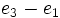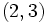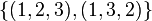# Modular representation theory of symmetric group:S3 at 2

View modular representation theory of particular groups | View other specific information about symmetric group:S3

This article describes the modular representation theory of symmetric group:S3 at the prime two, i.e., for fields of characteristic two, specifically field:F2 and its extensions.

For information on the linear representation theory in characteristic three (the other modular case) see modular representation theory of symmetric group:S3 at 3.

For information on the linear representation theory in other characteristics, see linear representation theory of symmetric group:S3.

## Summary

Item Value
degrees of irreducible representations (or equivalently, degrees of irreducible Brauer characters) 1,2
maximum: 2, lcm: 2, number: 2
smallest field of realization of irreducible representations in characteristic 2 field:F2, i.e., the field of two elements

## Family contexts

Family name Parameter values General description of modular representation theory of family
symmetric group degree$n = 3$ modular representation theory of symmetric groups at 2
special linear group of degree two over a finite field field:F2 modular representation theory of special linear group of degree two over a finite field in its defining characteristic

## Irreducible representations

There are two irreducible representations, both of them arising from irreducible representations in characteristic zero, namely:

Name of representation type Number of representations of this type Field of realization Kernel Degree Liftable to ordinary representation (characteristic zero)? How does the behavior differ from the non-modular case?
trivial 1 field:F2 whole group 1 Yes In the non-modular case, there are two one-dimensional representations, the trivial representation and the sign representation. Both of these reduce to the trivial representation in characteristic two.
standard (two-dimensional irreducible) 1 field:F2 trivial subgroup, i.e., it is faithful 2 Yes All the matrices now have determinant one, and in fact, the representation surjects to$SL(2,2)$, yielding$S_3 \cong SL(2,2)$.

### Trivial representation

The trivial or principal representation is a one-dimensional representation sending every element of the symmetric group to the identity matrix of order one.

### Standard representation

Further information: Standard representation of symmetric group:S3

This is obtained by computing the standard representation and then reducing modulo 2.Here is the description: consider a three-dimensional vector space with basis$e_1, e_2, e_3$. Let the symmetric group permute the basis vectors, and consider the induced action of the symmetric group on the vector space. This is a three-dimensional representation. Consider the two-dimensional subspace of all vectors of the form$x_1e_1 + x_2e_2 + x_3e_3$ where$x_1 + x_2 + x_3 = 0$. When the characteristic of the field is not two or three, this is a faithful, irreducible, two-dimensional representation. Note that$e_1 - e_2$ and$e_2 - e_3$ can be taken as a basis for this, with$e_3 - e_1$ being the negative of the sum of these.

The table below gives the matrices for the characteristic two case. Here, we convert all$-1$s to$1$s, because we are in characteristic two. Note that the map surjects to$SL(2,2) = GL(2,2)$.

Element Matrix for standard representation with basis$e_1 - e_2$,$e_2 - e_3$ Matrix for standard representation viewed as quotient with basis$\overline{e_1}, \overline{e_2}$ Characteristic polynomial Minimal polynomial Trace, character value
Identity element$\begin{pmatrix} 1 & 0 \\ 0 & 1 \\\end{pmatrix}$$\begin{pmatrix} 1 & 0 \\ 0 & 1 \\\end{pmatrix}$$x^2 + 1$$x + 1$ 0$(1,2,3)$$\begin{pmatrix} 0 & 1 \\ 1 & 1 \\\end{pmatrix}$$\begin{pmatrix} 0 & 1 \\ 1 & 1 \\\end{pmatrix}$$x^2 + x + 1$$x^2 + x + 1$ 1$(1,3,2)$$\begin{pmatrix} 1 & 1 \\ 1 & 0 \\\end{pmatrix}$$\begin{pmatrix} 1 & 1 \\ 1 & 0 \\\end{pmatrix}$$x^2 + x + 1$$x^2 + x + 1$ 1$(1,2)$$\begin{pmatrix} 1 & 1 \\ 0 & 1\\\end{pmatrix}$$\begin{pmatrix} 0 & 1 \\ 1 & 0 \\\end{pmatrix}$$x^2 + 1$$x^2 + 1$ 0$(2,3)$$\begin{pmatrix} 1 & 0 \\ 1 & 1 \\\end{pmatrix}$$\begin{pmatrix} 1 & 1 \\ 0 & 1 \end{pmatrix}$$x^2 + 1$$x^2 + 1$ 0$(1,3)$$\begin{pmatrix} 0 & 1 \\ 1 & 0\\\end{pmatrix}$$\begin{pmatrix} 1 & 0 \\ 1 & 1 \\\end{pmatrix}$$x^2 + 1$$x^2 + 1$ 0

## Character table

The character table (taking values in field:F2) is as follows:

Rep/Conj class$()$ ($2$-regular)$\{ (1,2,3), (1,3,2) \}$ ($2$-regular)$\{ (1,2), (1,3), (2,3) \}$ (not$2$-regular)
trivial 1 1 1
standard 0 1 0

## Brauer characters

Symmetric group:S3 is a rational representation group, in the sense that all its representations in characteristic zero can be realized using matrices with integer entries, and hence over the rational numbers. On the other side, all irreducible representations in characteristic two are realized over field:F2.

However, the eigenvalues of these representations do not live over field:F2, i.e., the matrices are not diagonalizable over this field. In order to diagonalize, we need to adjoin cube roots of unity on both sides. In other words, we need to fix a bijection between cube roots of unity in a quadratic extension of field:F2 and cube roots of unity in a quadratic extension of the rational numbers. Since the actual matrix entries and character values all live in the base fields, the Brauer characters ultimately will turn out not to depend on this choice of bijection.

### Brauer character table

There are two 2-regular conjugacy classes: the identity element and the 3-cycles. There are two Brauer characters: the character of the trivial representation and the character of the standard representation.

Irreducible representation in characteristic two whose Brauer character we are computing Irreducible representation in characteristic zero whose character equals the Brauer character Value of Brauer character on identity element Value of Brauer character on conjugacy class of$(1,2,3)$ and$(1,3,2)$
trivial representation trivial representation or sign representation 1 1
standard representation standard representation 2 -1

## Group ring interpretation

The group ring$\mathbb{F}_2[S_3]$ is not a semisimple module, and in particular, it does not break up as a direct product of matrix rings. In fact, the group ring$K[S_3]$ does not split up as a direct product of matrix rings even if we take$K$ to be the algebraic closure of$\mathbb{F}_2$.

## GAP implementation

The character degrees can be computed using GAP's CharacterDegrees function:

gap> CharacterDegrees(SymmetricGroup(3),2);
[ [ 1, 1 ], [ 2, 1 ] ]

The Brauer character table can be computed as follows:

gap> Irr(CharacterTable(SymmetricGroup(3),2));
[ Character( BrauerTable( Sym( [ 1 .. 3 ] ), 2 ), [ 1, 1 ] ),
Character( BrauerTable( Sym( [ 1 .. 3 ] ), 2 ), [ 2, -1 ] ) ]